Function Repository Resource:

# FunctionRootMeanSquare

Compute the root mean square of a function

Contributed by: Wolfram|Alpha Math Team
 ResourceFunction["FunctionRootMeanSquare"][expr,{var,a,b}] finds the root mean square of expr in terms of var over the interval from a to b. ResourceFunction["FunctionRootMeanSquare"][f,var] finds the root mean square of a periodic function f in terms of var.

## Details

The root mean square is the square root of the mean square, which is a special case of the generalized power mean. For a continuous function, the root mean square of a periodic function is the square root of the average value integral of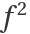over the the period. The root mean square (RootMeanSquare) also applies to lists of discrete values.

## Examples

### Basic Examples (3)

Compute the root mean square of cos(x) from 0 to 2π:

 In:=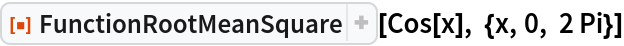Out=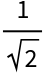Compute the root mean square for sin(x) over the function period:

 In:=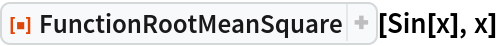Out=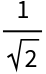Compute the root mean square for 3sin(t)-2cos(2t):

 In:=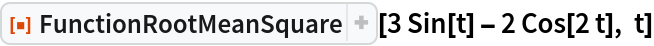Out=## Publisher

Wolfram|Alpha Math Team

## Version History

• 2.0.0 – 23 March 2023
• 1.0.0 – 11 January 2022

## Author Notes

To view the full source code for FunctionRootMeanSquare, evaluate the following:

 In:=